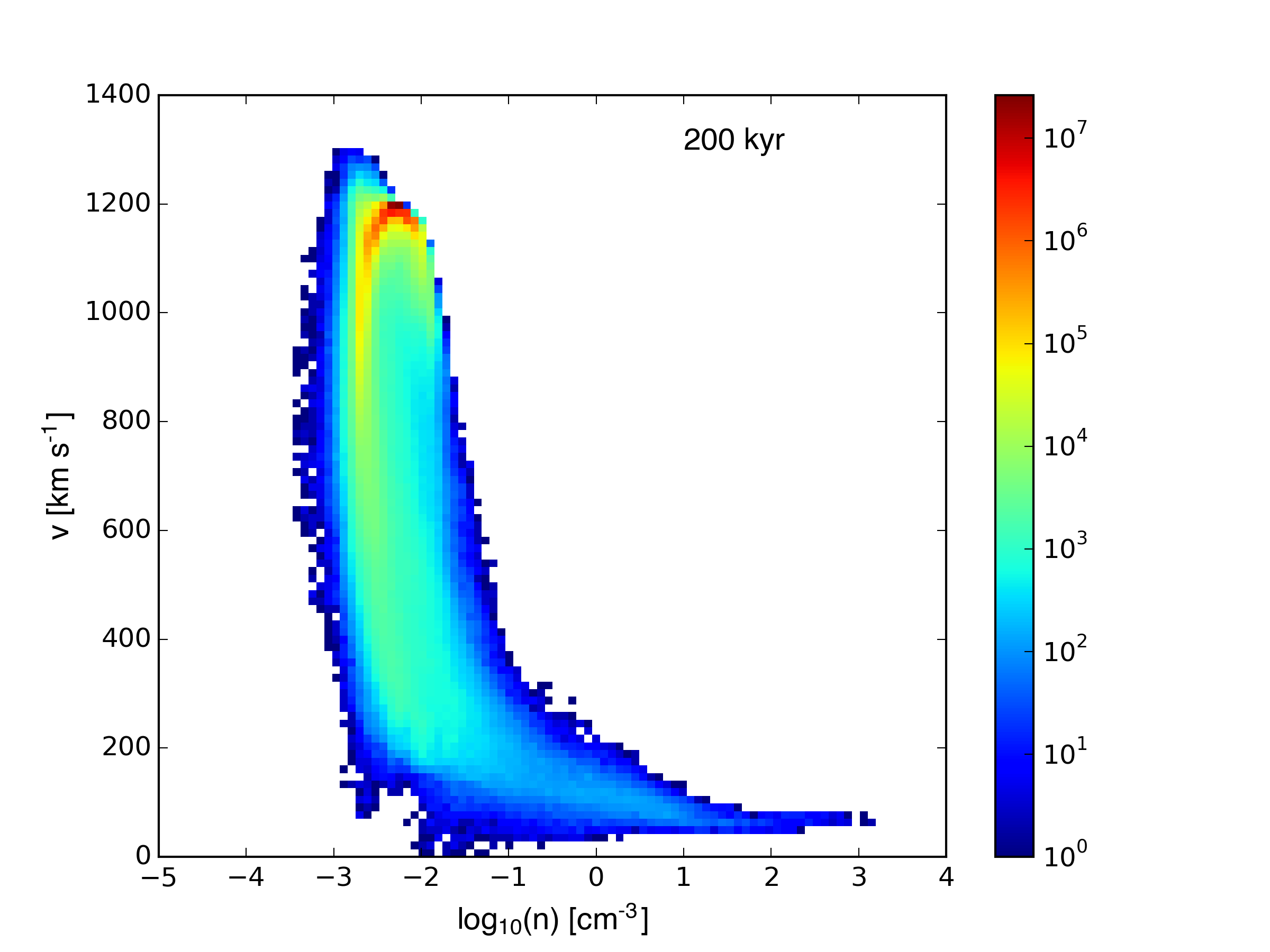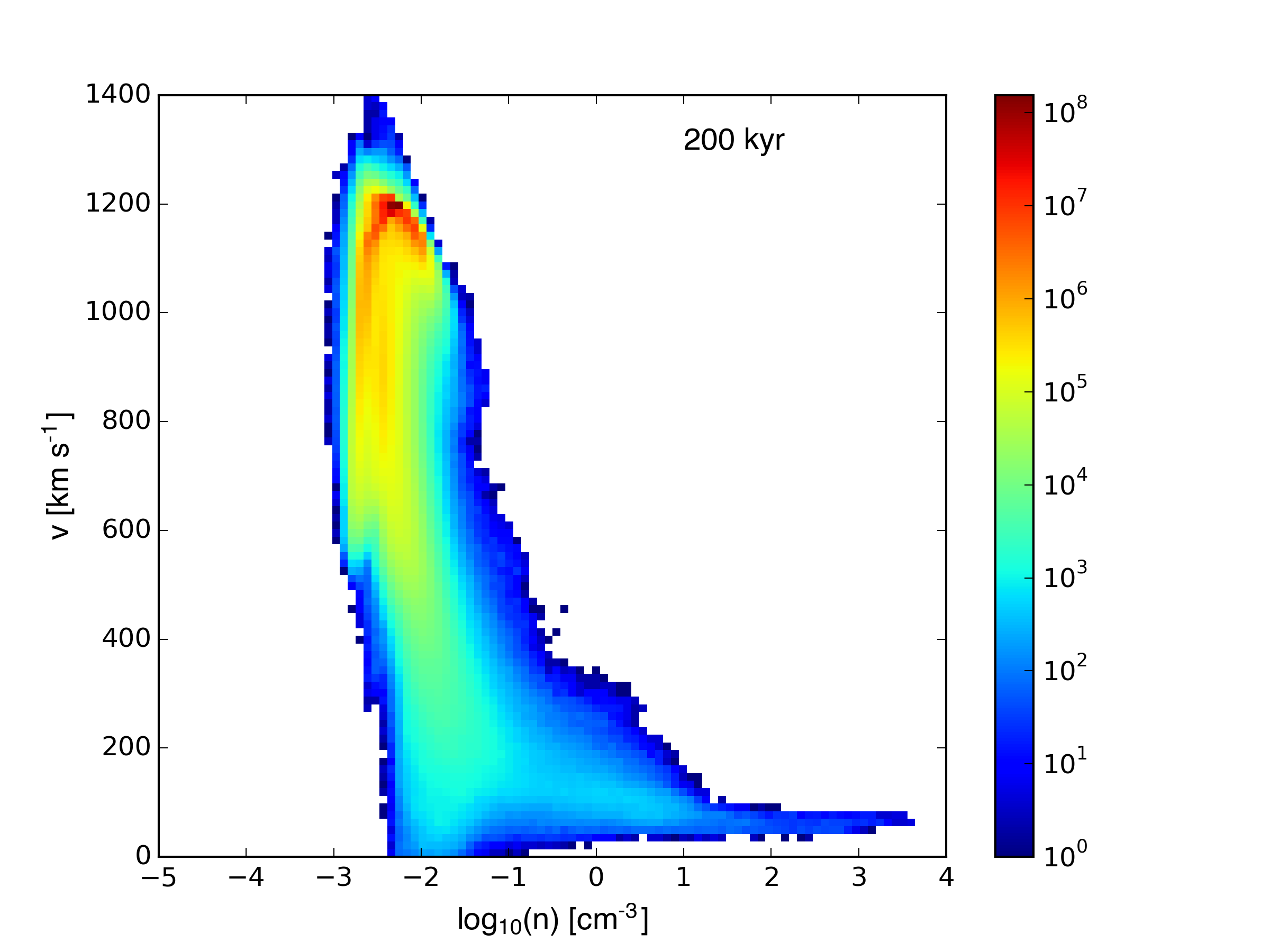Resolution Effects (Part 2)

April 4, 2016

The plots below compare the $n_h = 1$ sphere-wind simulation at two different resolutions. The low-resolution sim had 32 cells / $R_\mathrm{cloud}$, while the high-resolution sim had 64 cells / $R_\mathrm{cloud}$. Both simulations were run on a grid with physical size $160\times40\times40$ pc, but the high resolution had $2048\times512\times512$ cells, so the spatial resolution was 12.8 cells / parsec, vs 6.4 cells / parsec for the low resolution run. Both simulations use the new initial cloud density profile, which tapers exponentially to the wind density floor, starting at a cloud radius of 4.5 pc. The center of the cloud has a constant density. Both clouds have a mass of $\approx$ 10 solar masses.

Movies of both simulations are available: low resolution and high resolution.

Snapshots of the two clouds at 200, 400, 600, and 800 kyr: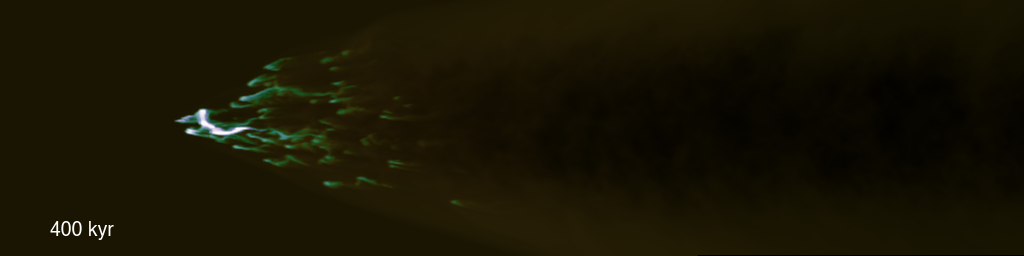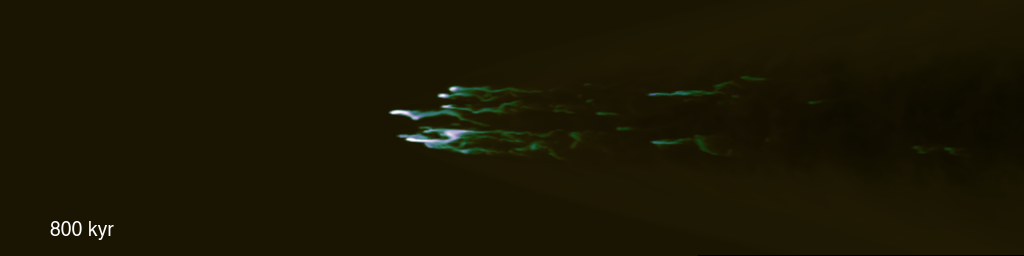Density-temperature plots (low res followed by high res):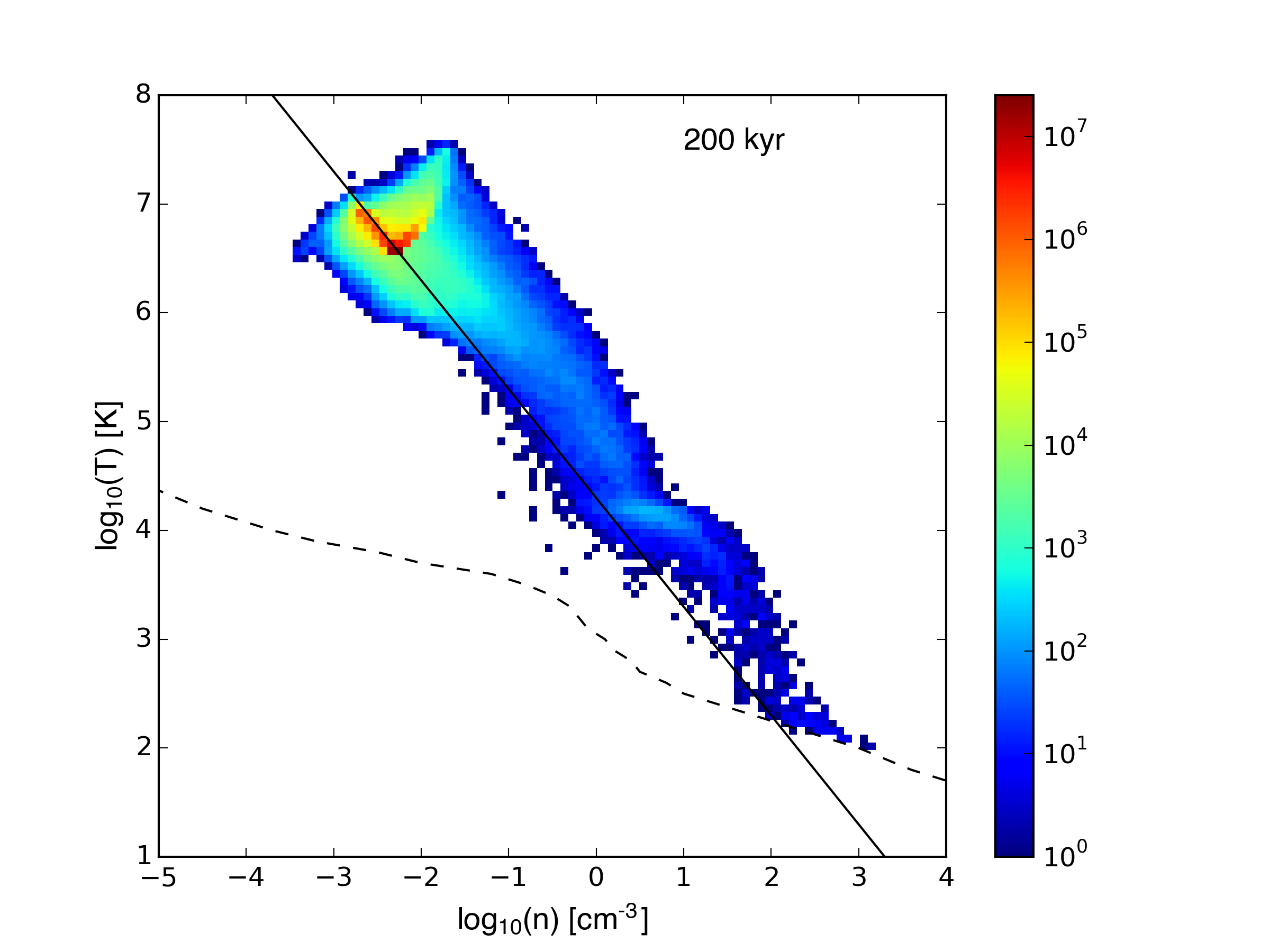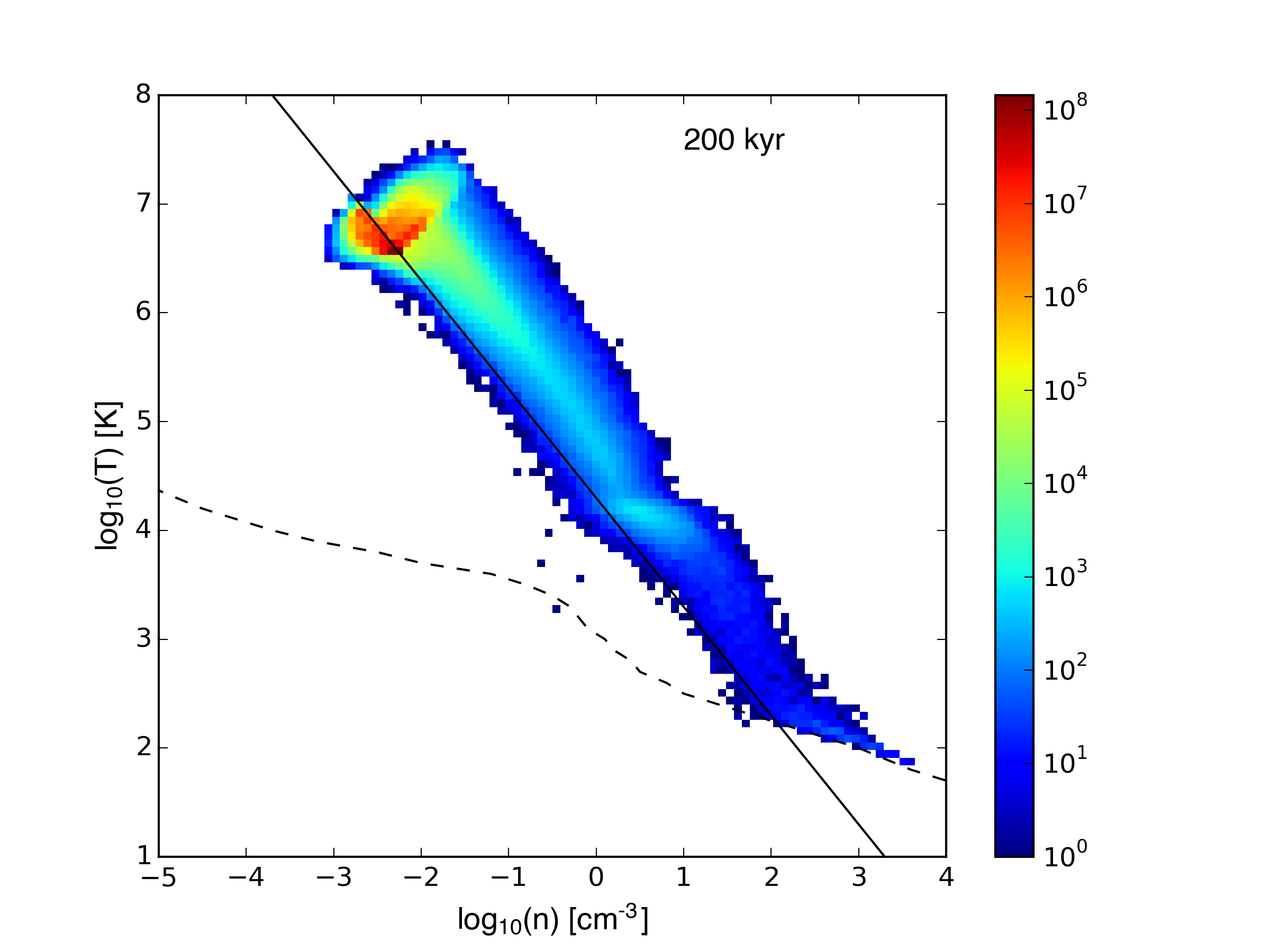Velocity-weighted density-temperature plots: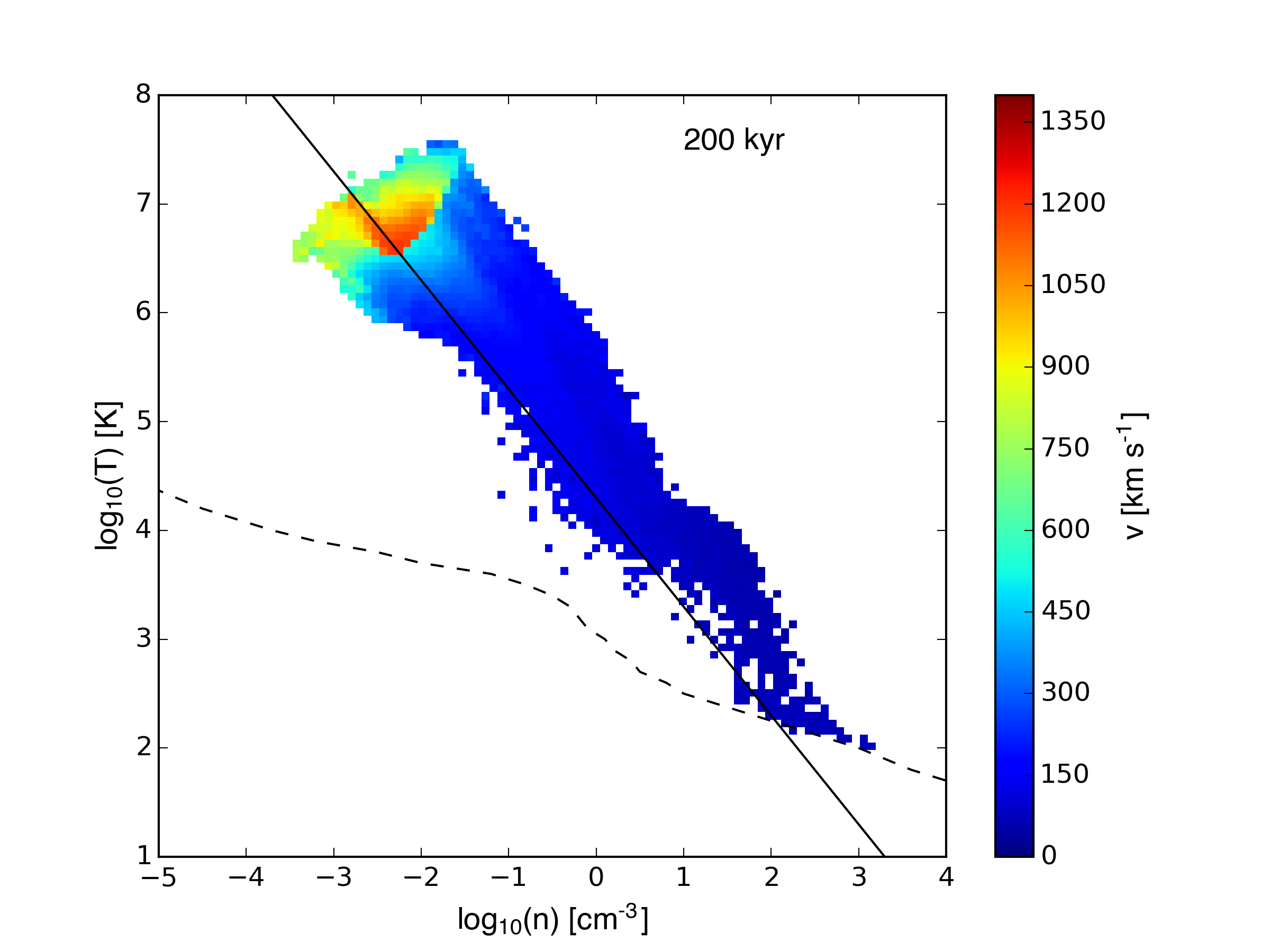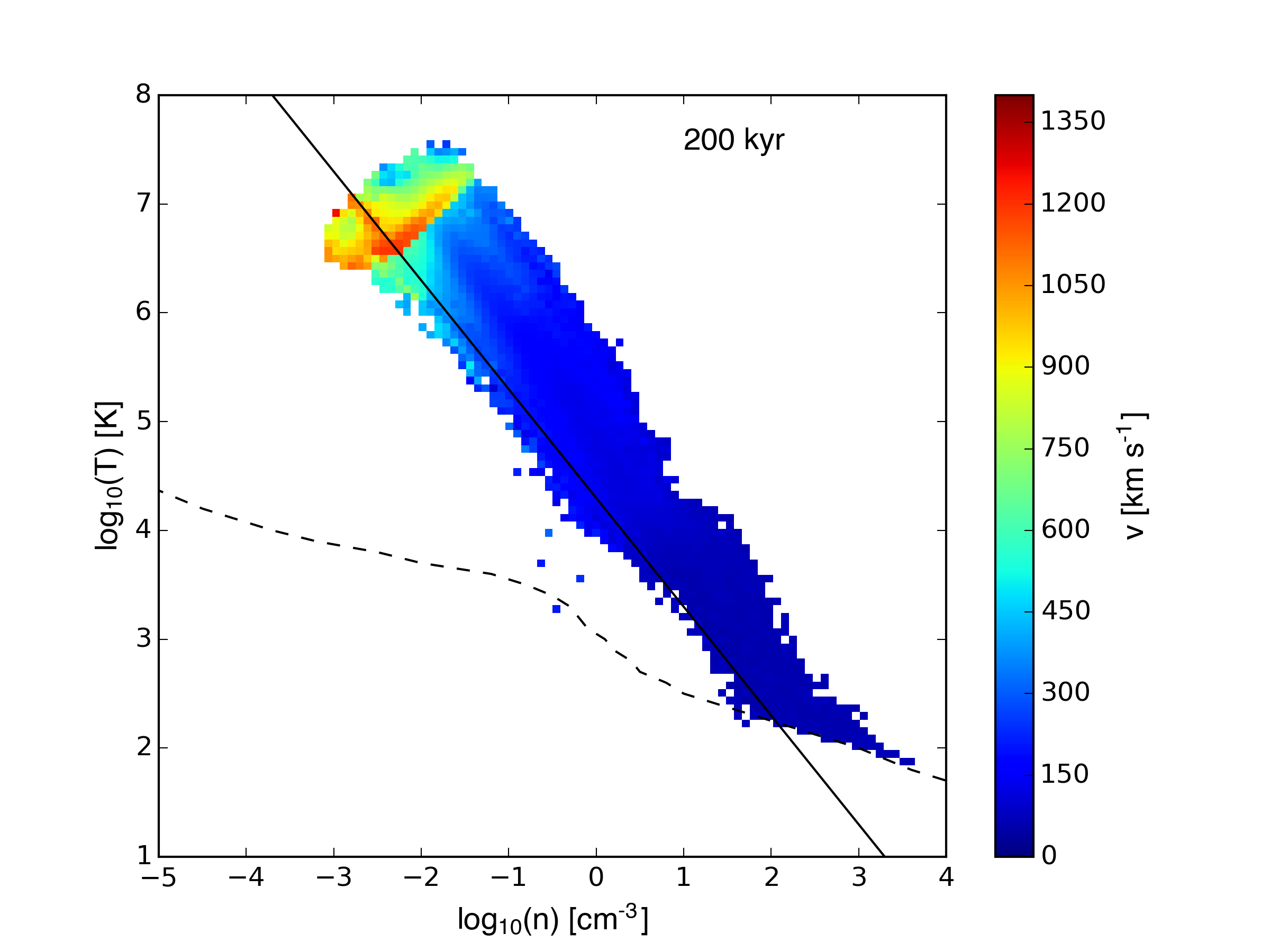Density-velocity plots: